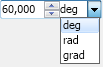﻿ Up Down Widgets
Nevron Open Vision Documentation
Up Down Widgets

Up down widgets allows the user to select a value from a specified range and generally consist of a widget (e.g. a text box), which shows the current value and a spinner that the user can use to increase/decrease the current value. The NOV provides 2 up down widgets:

Numeric Up Down

The numeric up down widget consists of a text box and a spinner with up and down buttons. The text box shows and also can be used to edit the current numeric value and accepts only digits and the decimal separator. The current value is stored in the Value property. When it changes the ValueChanged event is fired. The range is determined by the Minimum and Maximum properties. The Step property defines how much to increase/decrease the current value when the user clicks on the increase/decrease spinner button or presses the up/down key from the keyboard while the numeric up down text box is focused. You can also set the number of decimal places the numeric up down will show through the DecimalPlaces property.

The following example demonstrates how to create a numeric up down widget and subscribe for its ValueChanged event:

Numeric Up Down Example
Copy Code
```NNumericUpDown numericUpDown = new NNumericUpDown();
numericUpDown.Minimum = 0;
numericUpDown.Maximum = 100;
numericUpDown.Value = 40;
numericUpDown.ValueChanged += new Function<NValueChangeEventArgs>(OnNumericUpDownValueChanged);

private void OnNumericUpDownValueChanged(NValueChangeEventArgs arg1)
{
// Get the new value
double value = (double)arg1.NewValue;

// Get the numeric up down, whose value has changed
NNumericUpDown numericUpDown = (NNumericUpDown)arg1.CurrentTargetNode;
}
```
Measure Up Down

The measure up down extends the functionality of the numeric up down by adding a combo box for selecting a unit. If the units can be converted to each other, when the user selects a new unit from the unit combo box, the value will be automatically converted. The Unit property determines the currently selected measurement unit.

The following example demonstrates how to create a measure up down for angles:

Measure Up Down Example
Copy Code
```NMeasureUpDown measureUpDown = new NMeasureUpDown(NUnit.Degree, NUnit.Radian, NUnit.Grad);
measureUpDown.Value = 1;
measureUpDown.DecimalPlaces = 3;
measureUpDown.UnitComboBox.PreferredWidth = 40;
```

The result of the above code will be: